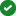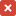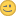# Different Result of calculation in view

XMLWordPrintable

#### Details

• Type:Bug
• Status: Confirmed
• Priority:Major
• Resolution: Unresolved
• Affects Version/s: 10.2, 10.3, 10.2.17, 10.3.9
• Fix Version/s:
• Component/s:
• Labels:
None
• Environment:

#### Description

After upgrading MariaDB an old view we are using suddenly returned different results. I have built an test case:

Two tables containing forms and position. Each form has n positions. Every position has an amount and every form has a discount, valid for all its positions:

 `CREATE TABLE `forms` (` ` `form_id` int(11) NOT NULL UNIQUE,` ` `discount` decimal(5,2) NOT NULL,` ` PRIMARY KEY (`form_id`)` `);` ` ` `CREATE TABLE `positions` (` ` `pos_id` int(11) NOT NULL AUTO_INCREMENT,` ` `form_id` int(11) NOT NULL,` ` `amount` decimal(9,2) NOT NULL,` ` PRIMARY KEY (`pos_id`)` `);` ` ` `INSERT INTO `forms` (`form_id`, `discount`) VALUES ('1', '10');` ```INSERT INTO `positions` (`pos_id`, `form_id`, `amount`) VALUES (NULL, '1', '100'), (NULL, '1', '200'); ```

When I query this, I get the right result:
100 + 200 = 300
-10% = 270

 `SELECT` ` F.form_id,` ` COUNT(P.pos_id) AS pos,` ` SUM(P.amount) * (100 - F.discount)/100 AS total` `FROM `forms` AS F` ` INNER JOIN `positions` AS P USING(form_id)` ```GROUP BY form_id; ```

 Output `+---------+-----+--------------+` `| form_id | pos | total |` `+---------+-----+--------------+` `| 1 | 2 | 270.00000000 |` `+---------+-----+--------------+` ```1 row in set (0.001 sec) ```

Now when I wrap exactly this SQL statement inside an view, a different result occurs:

 `CREATE VIEW view_1 AS` `SELECT` ` F.form_id,` ` COUNT(P.pos_id) AS pos,` ` SUM(P.amount) * (100 - F.discount)/100 AS total` `FROM `forms` AS F` ` INNER JOIN `positions` AS P USING(form_id)` `GROUP BY form_id;` ` ` ```SELECT * FROM view_1; ```

 Output `+---------+-----+--------------+` `| form_id | pos | total |` `+---------+-----+--------------+` `| 1 | 2 | 29999.900000 |` `+---------+-----+--------------+` ```1 row in set (0.001 sec) ```

Problem is obviously, that the parentheses in SUM(P.amount) * (100 - F.discount)/100 are ignored in the VIEW and therefor the multiplication is executed before the subtraction.

 w/ parentheses `SUM(P.amount) * (100 - F.discount)/100` `= (100+200) * (100 - 10 )/100` `= 300 * 90/100` `= 300 * 0.9` ```= 270 ```

 w/o parentheses `SUM(P.amount) * 100 - F.discount/100` `= (100+200) * 100 - 10/100` `= 300 * 100 - 0.9` ```= 29999.9 ```

The problem occurred when upgrading a docker container to mariadb:latest. Unfortunately I cannot say which version we were running before.

So I have tested all the latest minor releases of 5.5 and 10.x from the official docker repo:

Version Image Works as expected
5.5.54 5.5.54-MariaDB-1~wheezy10.0.36 10.0.36-MariaDB-1~xenial10.1.36 10.1.36-MariaDB-1~bionic10.2.17 10.2.17-MariaDB-1:10.2.17+maria~bionic10.3.9 10.3.9-MariaDB-1:10.3.9+maria~bionicHope that helps. It's my first bug here#### People

Assignee:Oleksandr Byelkin
Reporter:Björn Gießler